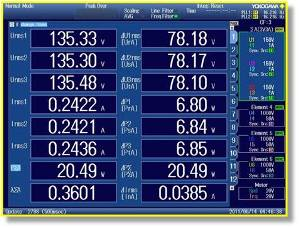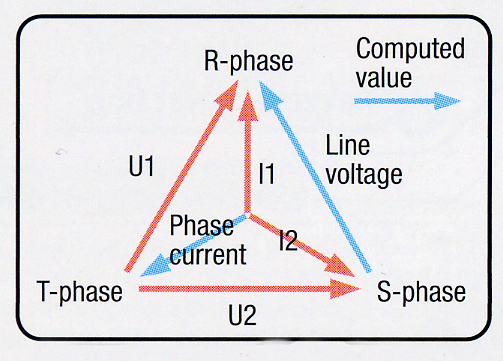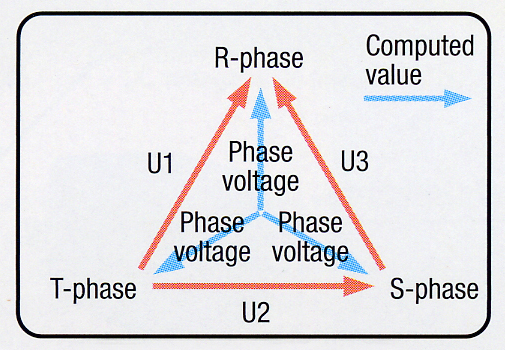# Delta Computation Function

It is possible to obtain the differential voltage, line voltage, phase voltage, etc. by obtaining the sums and differences of instantaneous measurement values of voltage and current in each element.

• Differential voltage/current: Differential voltage and current between two elements are computed in the three-phase three-wire system.
• Line voltage/phase current: Line voltage and phase current that are not measured are computed in the three-phase three-wire system (Figure 1).
• Star-delta conversion: Line voltage is computed from the phase voltage using the three-phase four-wire system data.
• Delta-star conversion: Phase voltage is computed from the line voltage in the three-phase three-wire system (3V3A system)
(Figure 2).Figure 1. Line voltage/phase currentFigure 2. Delta-star conversion

### Related Products & Solutions

#### WT1800E - High-Performance

• Power, Harmonics (THD) Measurements (up to 500th order)
• Electrical & Mechanical Efficiency of Inverters and Motors in EV and HEV

Top